Abstract

We describe and analyze a simple and effective iterative algorithm for solving the optimization problem cast by Support Vector Machines (SVM). Our method alternates between stochastic gradient descent steps and projection steps. We prove that the number of iterations required to obtain a solution of accuracy is . In contrast, previous analyses of stochastic gradient descent methods require iterations. As in previous devised SVM solvers, the number of iterations also scales linearly with , where is the regularization parameter of SVM. For a linear kernel, the total run-time of our method is , where is a bound on the number of non-zero features in each example. Since the run-time does not depend directly on the size of the training set, the resulting algorithm is especially suited for learning from large datasets. Our approach can seamlessly be adapted to employ non-linear kernels while working solely on the primal objective function. We demonstrate the efficiency and applicability of our approach by conducting experiments on large text classification problems, comparing our solver to existing state-of-the art SVM solvers. For example, it takes less than 5 seconds for our solver to converge when solving a text classification problem from Reuters Corpus Volume 1 (RCV 1) with training examples.# 1. Introduction

Support Vector Machines (SVMs) are effective and popular classification learning tool. The task of learning a support vector machine is cast as a constrained quadratic programming. However, in its native form, it is in fact an unconstrained empirical loss minimization with a penalty term for the norm of the classifier that is being learned. Formally, given a training set , where and , we would like to find the minimizer of the problem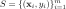(1)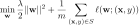where

(1)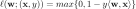We denote the objective function of Eq. (1) by . An optimization method finds an -accurate solution if . The original SVM problem also includes a bias term, . We omit the bias throughout the first sections and defer the description of an extension which employs a bias term to Sec. 4.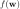We describe and analyze in this paper a simple iterative algorithm, called Pegasos, for solving Eq. (1). The algorithm performs iterations and also requires an additional parameter , whose role is explained in the sequel. Pegasos alternates between stochastic subgradient descent steps and projection steps. The parameter determines the number of examples from the algorithm uses on each iteration for estimating the subgradient. When , Pegasos reduces to a variant of the subgradient projection method. We show that in this case the number of iterations that is required in order to achieve an -accurate solution is . At the other extreme, when , we recover a variant of the stochastic (sub) gradient method. In the stochastic case, we analyze the probability of obtaining a good approximate solution. Specifically, we show that with probability of at least our algorithm finds an -accurate solution using only iterations, while each iteration involves a single inner product between and . This rate of convergence does not depend on the size of the training set and thus our algorithm is especially suited for large datasets.# 2. The Pegasos Algorithm

In this section we describe the Pegasos algorithm for solving the optimization problem given in Eq. (1). The algorithm receives as input two parameters: - the number of iterations to perform; - the number of examples to use for calculating sub-gradients. Initially, we set to any vector whose norm is at most . On iteration of the algorithm, we first choose a set of size . Then, we replace the objective in Eq. (1) with an approximate objective function,.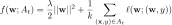Note that we overloaded our original definition of as the original objective can be denoted either as or as . We interchangeably use both notations depending on the context. Next, we set the learning rate and define to be the set of examples for which suffers a non-zero loss. We now perform a two-step update as follows. We scale by and for all examples we add to the vector . We denote the resulting vector by . This step can be also written as , where(1)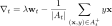The definition of the hinge-loss implies that is a sub-gradient of at . Last, we set to be the projection of onto the set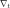(1)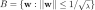That is, is obtained by scaling by . As we show in our analysis below, the optimal solution of SVM is in the set . Informally speaking, we can always project back onto the set as we only get closer to the optimum. The output of Pegasos is the last vector .Note that if we choose on each round then we obtain the sub-gradient projection method. On the other extreme, if we choose to contain a single randomly selected example, then we recover a variant of the stochastic gradient method. In general, we allow to be a set of examples sampled i.i.d. from .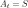We conclude this section with a short discussion of implementation details when the instances are sparse, namely, when each instance has very few non-zero elements. In this case, we can represent as a triplet where is a dense vector and are scalars. The vector is defined through the triplet as follows: and stores the squared norm of , . Using this representation, it is easily verified that the total number of operations required for performing one iteration of Pegasos with is , where is the number of non-zero elements in .# 3. Analysis

In this section we analyze the convergence properties of Pegasos. Throughout this section we denote

(1)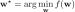Recall that on each iteration of the algorithm, we focus on an instantaneous objective function

## Pegasos: Primal Estimated sub-GrAdient Solver for SVM的更多相关文章

1. [转] 从零推导支持向量机 (SVM)

原文连接 - https://zhuanlan.zhihu.com/p/31652569 摘要 支持向量机 (SVM) 是一个非常经典且高效的分类模型.但是,支持向量机中涉及许多复杂的数学推导,并需要 ...

2. 损失函数(Loss Function) -1

http://www.ics.uci.edu/~dramanan/teaching/ics273a_winter08/lectures/lecture14.pdf Loss Function 损失函数 ...

3. 常见数据挖掘算法的Map-Reduce策略(2)

接着上一篇文章常见算法的mapreduce案例(1)继续挖坑,本文涉及到算法的基本原理,文中会大概讲讲,但具体有关公式的推导还请大家去查阅相关的文献文章.下面涉及到的数据挖掘算法会有:L ...

4. 逻辑回归原理_挑战者飞船事故和乳腺癌案例_Python和R_信用评分卡（AAA推荐）

sklearn实战-乳腺癌细胞数据挖掘(博客主亲自录制视频教程) https://study.163.com/course/introduction.htm?courseId=1005269003&a ...

5. 基于MNIST数据的softmax regression

跟着tensorflow上mnist基本机器学习教程联系 首先了解sklearn接口: sklearn.linear_model.LogisticRegression In the multiclas ...

6. [Scikit-learn] 1.1 Generalized Linear Models - Logistic regression &amp; Softmax

二分类:Logistic regression 多分类:Softmax分类函数 对于损失函数,我们求其最小值, 对于似然函数,我们求其最大值. Logistic是loss function,即: 在逻 ...

7. Factorization Machine算法

参考: http://stackbox.cn/2018-12-factorization-machine/ https://baijiahao.baidu.com/s?id=1641085157432 ...

9. SVM应用

我在项目中应用的SVM库是国立台湾大学林智仁教授开发的一套开源软件,主要有LIBSVM与LIBLINEAR两个,LIBSVM是对非线性数据进行分类,大家也比较熟悉,LIBLINEAR是对线性数据进行分 ...

## 随机推荐

1. Stored Procedure 里的 WITH RECOMPILE 到底是干麻的？

在 SQL Server 创建或修改「存储过程(stored procedure)」时,可加上 WITH RECOMPILE 选项,但多数文档或书籍都写得语焉不详,或只解释为「每次执行此存储过程时,都 ...

2. SQLServer：删除log文件和清空日志的方法

数据库的性能是DBA都需要重点关注的,日志文件的增多严重影响数据库的性能,本文将为您介绍SQL Server删除日志文件的方法,供您参考,希望对您有所帮助. 数据库在使用过程中会使日志文件不断增加,使 ...

3. Java for LeetCode 205 Isomorphic Strings

Given two strings s and t, determine if they are isomorphic. Two strings are isomorphic if the chara ...

4. iOS.ReactNative-4-react-native-command-line-tool

Command line tool: react-native 1. react-native 是一个命令行工具 1.1 react-native简介 运行以下命令: ls -lt `which re ...

5. html之label标签

label标签为input元素定义标注,label标签与相关元素通过id属性绑定在一起. 相关属性: for:规定label绑定到哪个表单元素 form:规定label字段所属的一个或多个表单 示例代 ...

http://site.douban.com/129642/widget/notes/5513129/note/187701199/ 1-view controllers的关系:Each custom ...

7. 自然语言处理(5)之Levenshtein最小编辑距离算法

自然语言处理(5)之Levenshtein最小编辑距离算法 题记:之前在公司使用Levenshtein最小编辑距离算法来实现相似车牌的计算的特性开发,正好本节来总结下Levenshtein最小编辑距离 ...

8. poj 3294 Life Forms - 后缀数组 - 二分答案

题目传送门 传送门I 传送门II 题目大意 给定$n$个串,询问所有出现在严格大于$\frac{n}{2}$个串的最长串.不存在输出'?' 用奇怪的字符把它们连接起来.然后求sa,hei,二分答案,按 ...

9. python拓展1 week1-week5复习回顾

知识内容: 1.python基础概念及基础语法 2.python基础数据类型 3.python模块相关 4.python函数相关 5.python面向对象相关 6.python文件处理相关 注:本节内 ...

10. jstorm知识整理

最近在做一个jstorm的程序.我的jstorm程序消费一个kafka主题,根据数据逻辑判断需要往下游哪几个kafka主题的生产者发送. 1.bolt的execute(Tuple input)方法每次 ...